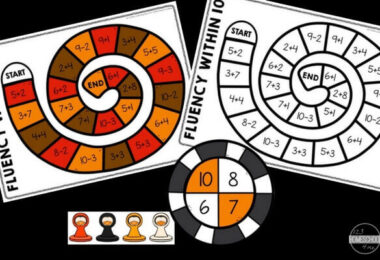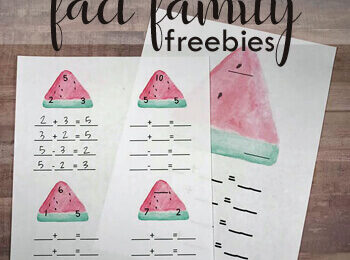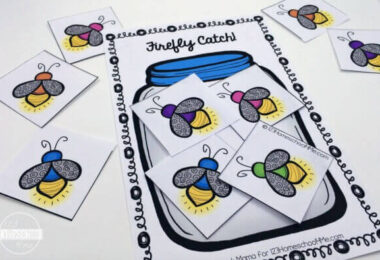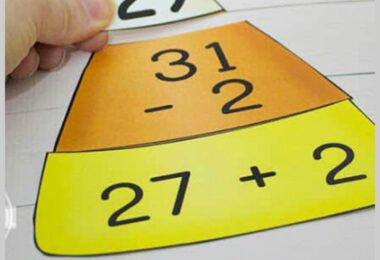Category - Subtraction

So many fun subtraction activities, subraction worksheets, and subtractin games …. which FREE subtraction resource will you try first?

These are the perfect addition to any math curriculum to add in some extra subtraction practice to achieve math fluency that is so important for future math. Repeated math practice is key to accuracy and speed for kindergarten math, grade 1 math, grade 2 math, grade 3 math, and grade 4 math.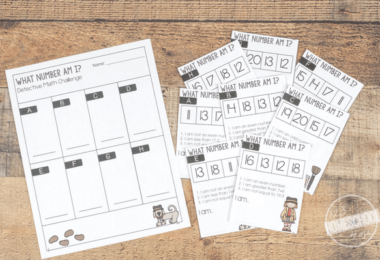Addition and Subtraction Math Mystery Printable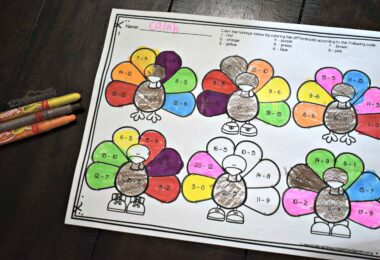Turkey Subtraction – Thanksgiving Math Worksheets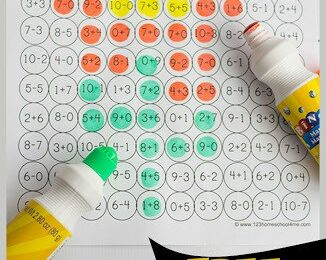Solve and Stamp Addition and Subtraction Worksheets with Dot...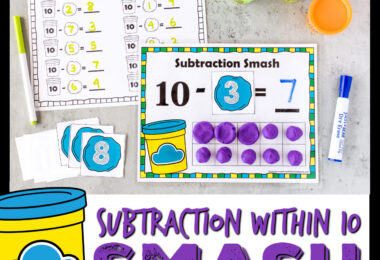FREE Printable Playdough Subtraction Smash Math Activity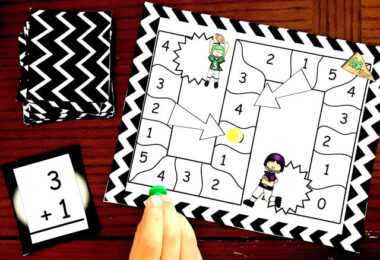FREE Addition and Subtraction Games Printable within 5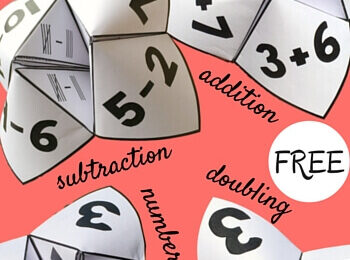FREE Printable Cootie Catchers Math Games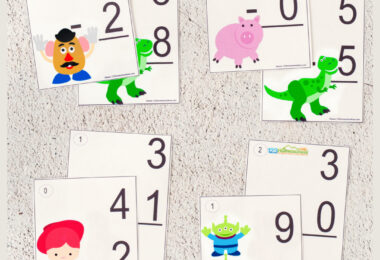FREE Printable Toy Story Subtraction Flashcards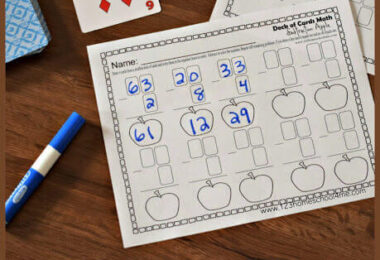Deck of Cards Subtraction Worksheets Make Practice FUN!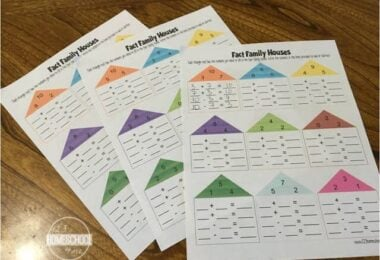FREE Fact Family Worksheets for Kindergarten and 1st Grade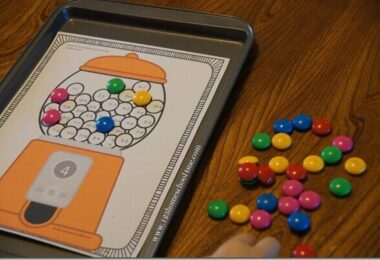Gumball Math Worksheets – Addition and Subtraction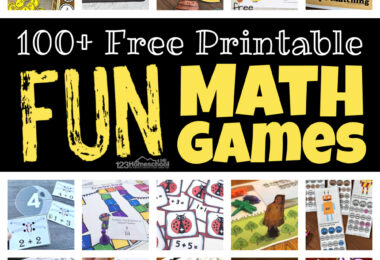FUN Printable Math Games for Elementary Age Kids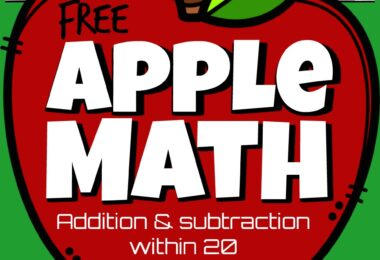Kindergarten Apple Math Worksheets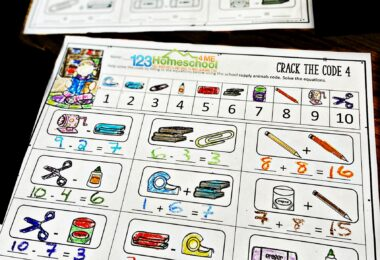Back to School Crack the Code Worksheets for First Day Activity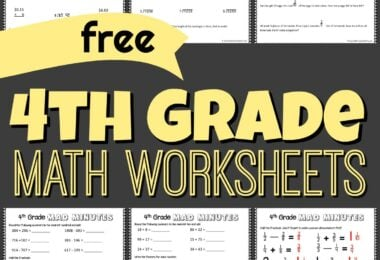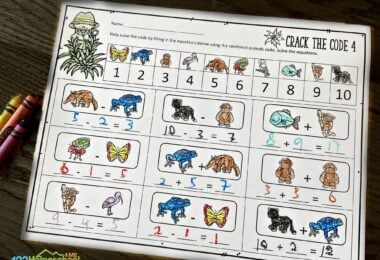Crack the Code Kindergarten Addition Math Worksheet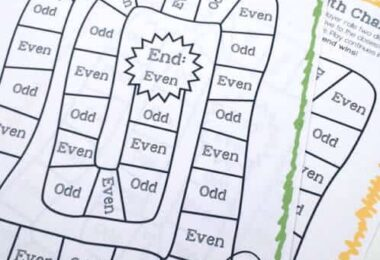Free Printable Summer Math Games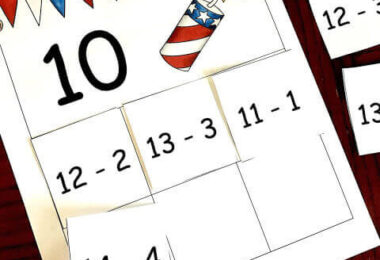4th of July Math – Patriotic Subtraction Mats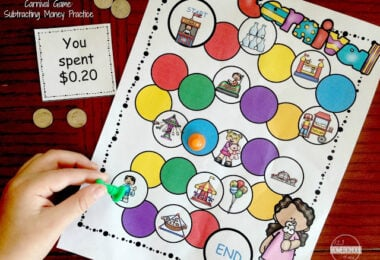Carnival Subtracting Money Games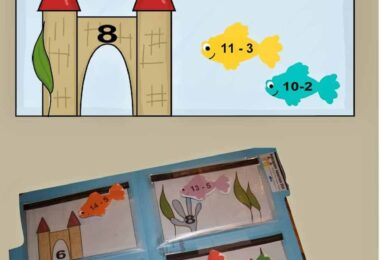Aquarium Subtraction Games for Grade 1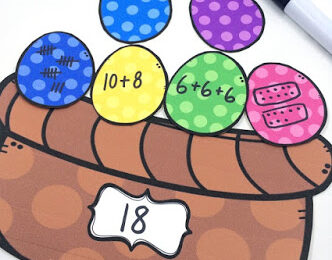Easter Basket Math Challenge w/ FREE Printables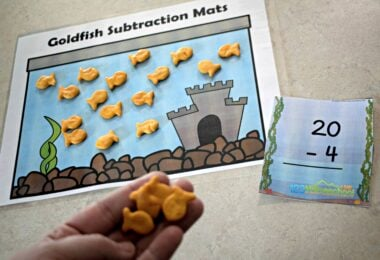Goldfish Kindergarten Subtraction Game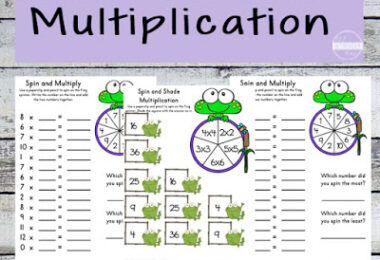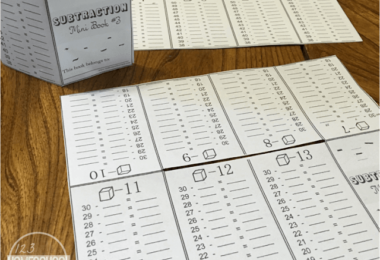Subtraction Mini-Book Worksheets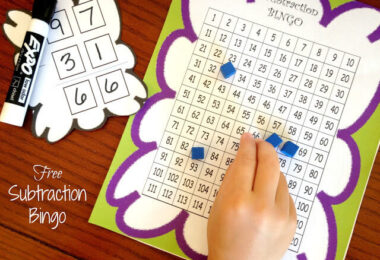Subtraction Bingo Math Game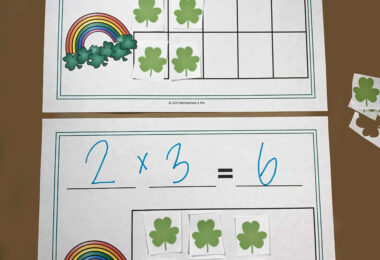FREE St Patrick’s Day Math Equation Mats Printable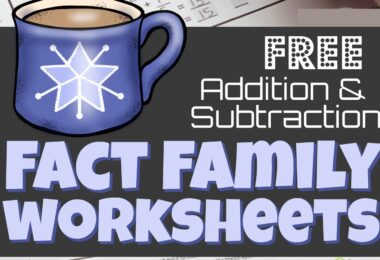Hot Chocolate Addition and Subtraction Fact Family Worksheets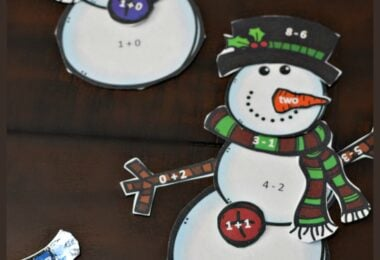Snowman Math – Practice Addition and Subtraction to 10...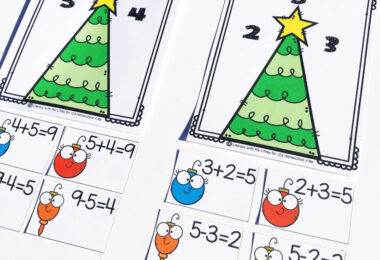Christmas Fact Families Activity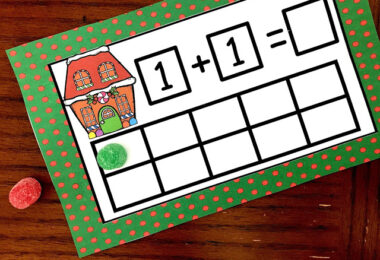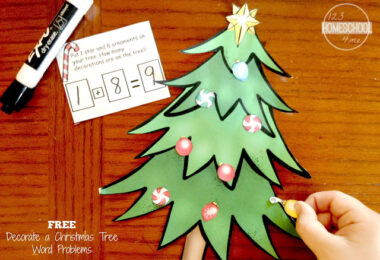Christmas Word Problems – Decorate the Tree with math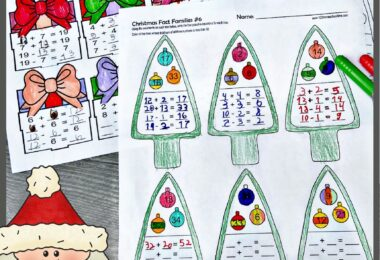Christmas Fact Families – Addition and Subtraction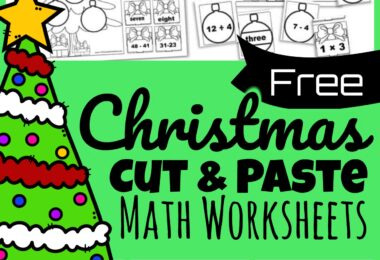Christmas Math Worksheets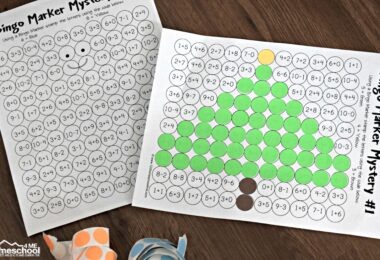Solve and Stamp Christmas Math Worksheets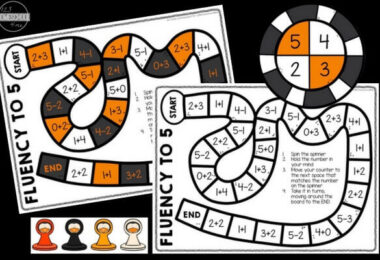Fluency within 5 Addition and Subtraction Games